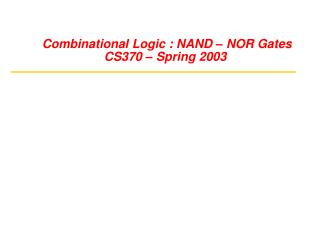# Combinational Logic : NAND – NOR Gates CS370 – Spring 2003 - PowerPoint PPT PresentationDownload PresentationCombinational Logic : NAND – NOR Gates CS370 – Spring 2003

Combinational Logic : NAND – NOR Gates CS370 – Spring 2003
Download Presentation## Combinational Logic : NAND – NOR Gates CS370 – Spring 2003

- - - - - - - - - - - - - - - - - - - - - - - - - - - E N D - - - - - - - - - - - - - - - - - - - - - - - - - - -
##### Presentation Transcript

1. Multi-Level Logic: Advantages Reduced sum of products form: x = A D F + A E F + B D F + B E F + C D F + C E F + G 6 x 3-input AND gates + 1 x 7-input OR gate (may not exist!) 25 wires (19 literals plus 6 internal wires) Factored form: x = (A + B + C) (D + E) F + G 1 x 3-input OR gate, 2 x 2-input OR gates, 1 x 3-input AND gate 10 wires (7 literals plus 3 internal wires)

2. A A B B A + B A · B A + B A · B 0 1 0 1 0 0 1 1 0 1 1 0 1 1 1 1 1 0 0 1 1 1 1 1 1 0 1 0 1 1 0 0 Multi-Level Logic: Conversion of Forms NAND-NAND and NOR-NOR Networks DeMorgan's Law: (A + B)' = A' · B'; (A ·B)' = A' + B' Written differently: A + B = (A' · B')'; (A · B) = (A' + B')' In other words, OR is the same as NAND with complemented inputs AND is the same as NOR with complemented inputs NAND is the same as OR with complemented inputs NOR is the same as AND with complemented inputs OR/NAND Equivalence A A OR OR B B A A Nand Nand B B

3. A A B B A · B A + B A · B A + B 0 1 0 1 0 0 1 1 0 1 1 0 0 0 0 0 1 0 0 1 0 0 0 0 1 0 1 0 1 1 0 0 Mult-Level Logic: Conversion Between Forms AND/NOR Equivalence A A AND AND B B A A NOR NOR B B Introduce appropriate inversions(“bubbles”)to convert from networks with ANDs and ORs to networks with NANDs and NORs. Each introduced "bubble" must be matched with a corresponding "bubble“ to preserve logic levels

4. Multi-Level Logic: Conversion of Forms Example: Map AND/OR network to NAND/NAND network (A) (B) AND OR AND NAND (C) (D) NAND NAND NAND NAND NAND

5. Multi-Level Logic: Conversion of Forms Example: Map AND/OR network to NAND/NAND network NAND NAND A A NAND B B Z Z C C D D Z = [(A · B)' + (C · D)']' = [(A' + B')+ (C' + D')]' = [(A' + B')' ·(C' + D')'] = (A · B) + (C · D) Equivalence of the two forms can be verified This is the easy conversion!

6. Multi-Level Logic: Mapping Between Forms Example: Map AND/OR network to NOR/NOR network A \A NOR NOR \B NOR B Z Z C \C NOR NOR D \D Step 2 Step 1 Conserve "Bubbles" Conserve "Bubbles" Z = {[(A' + B')' + (C' + D')']'}' = {(A' + B') ·(C' + D')}' = (A' + B')' + (C' + D')' = (A · B) + (C · D) Equivalence of the two forms can be verified This is the hard conversion! AND/OR to NAND/NAND more natural

7. Multi-Level Logic: Mapping Between Forms Example: Map OR/AND network to NOR/NOR network A A NOR B B NOR C C NOR D D Conserve Bubbles Z = [(A + B)' + (C + D)']' = {(A + B)'}' · {(C + D)'}' = (A + B) · (C + D) Equivalence of the two forms Can be verified This is the easy conversion!

8. Multi-Level Logic: Mapping Between Forms Example: Map OR/AND network to NAND/NAND network Nand Nand Nand Nand Nand Step 2 Step 1 Conserve Bubbles! Conserve Bubbles! {[(A' ·B')' · (C' ·D')']'}' = {(A' ·B') + (C' ·D')}' = (A' ·B')' · (C' ·D')' = (A + B) ·(C + D) Z = Verify equivalence of the two forms OR/AND to NOR/NOR more natural This is the hard conversion!

9. Add double bubbles at inputs Original circuit Distribute bubbles some mismatches Insert inverters to fix mismatches Multi-Level Logic: More than Two-Levels Conversion Example